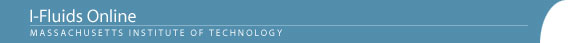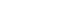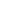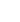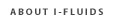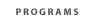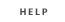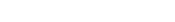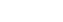2.25: Advanced Fluid Dynamics Section 1: Continuum viewpoint and the equation of motion Section 2: Static Fluids Section 3: Mass Conservation Section 4: Inviscid flow - differential approach Section 5: Control Volume Theorums Section 6: Navier-Stokes equation and viscous flow Section 7: Similarity and dimensional analysis Section 8: Boundary layers, separation and effect on drag/lift Section 9: Vorticity and circulation Section 10: Potential flows; lift, drag and thrust production Section 11: Surface tension and its effect on flows Section 12: Introduction to turbulence Back to 2.25 HomeSection 11: Surface Tension11.1 Surface tension—its molecular origin and its characterization by means of a surface tension coefficient based on either energy (s=energy/area) or mechanical (s=force/length) concepts. Simple derivation of the Young-Laplace equation for the pressure jump at the surface of a spherical liquid drop, based on energy considerations as well as force considerations. Generalization to arbitrary bounding surfaces.
11.2 Drops and bubbles. Effect of gravity: the Bond number. Effect of flow: the Weber number (for Re>>1) and the Capillary number (for Re<1).
11.3 The contact angle - an "equilibrium property" of a line of separation between a solid and two immiscible fluids. Wetting and non-wetting conditions. Advancing and receding contact angles; contact angle hysterisis. Young's equation for the contact angle in terms of the interfacial energies associated with the three phases (derived from either a force balance or energy minimization).
11.4 Equilibrium capillary rise derived from either (i) pressures distribution, (ii) control volume, or (iii) energy minimization. Examples: Wicking; sap in trees rising to leaves (where it evaporates); startup of flow through a pinhole in bottom of a bucket being filled.
11.5 Thickness of liquid puddles at equilibrium on a solid horizontal surface. (Solution by control volume method as well as energy method). Capillary rise or fall of liquid level adjacent to a vertical wall.
11.6 Adhesion or repulsion between partially wetted solid surfaces. (Why wet plates stick together, why one can pick up grains of sand with a wetted finger, etc.)
11.7 Attraction or repulsion of bodies touching or penetrating a liquid surface. (The pond-skater and other examples from insect life.)
11.8 Breakup of a small coherent jet into a droplet stream (atomization).
11.9 Breakup of larger drops moving through fluid. Drop impact (and breakup) on solid surfaces.
11.10 Effect of surface tension gradients on the interfacial boundary condition (the Marangoni effect). The dependence on surface tension on temperature and surfactant concentration. Examples: Immobilization of the surface of small air bubbles rising through a seawater. What prevents the liquid in a soap bubble from simply falling down? The calming effect of oil on wind waves on water.
11.11 Equilibrium and non-equilibrium conditions at lines of contact between three immiscible fluids. Spreading of oil slicks on water.
11.12 Capillary waves on water.

Reading
Fay. pages 11-13, 53-55
General reference: A. W. Adamson, Physical Chemistry of Surfaces, Wiley

Problem Set Section 11

Problem 2.2
Problem 2.5
Problem 2.6
Problem 2.7